# HandCalcs module in Python

• Last Updated : 05 Sep, 2020

HandCalcs is a library in Python to make calculations automatically in Latex, but in a way that imitates how the equation might be formatted when handwritten, write the mathematical formula, supported by numerical substitutions, and then the output. Since HandCalcs indicates the numerical replacement, the equations become much easier to manually check and verify.

### Installation

Run the following pip command on the terminal.

Attention geek! Strengthen your foundations with the Python Programming Foundation Course and learn the basics.

To begin with, your interview preparations Enhance your Data Structures concepts with the Python DS Course. And to begin with your Machine Learning Journey, join the Machine Learning - Basic Level Course

```pip install handcalcs
```

The HandCalcs library in Python is designed to be used in Jupyter Notebook or Jupyter Lab as a cell magic.
To use the render feature of the HandCalcs library, import the module by executing import handcalcs.render, after that just use the %%render on the top of the cell in which equations or variables are to be rendered with HandCalcs.

Example 1: Addition of 2 numbers

## Python3

 `# importing the module``import` `handcalcs.render`` ` `x ``=` `5``y ``=` `6`` ` `# run the code below in a new Jupyter cell``%``%``render``z ``=` `x ``+` `y`

Output: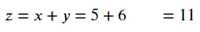Example 2: Calculating the tan of an expression.

## Python3

 `# importing the libraries``import` `handcalcs.render``from` `math ``import` `tan`` ` `p ``=` `5``r ``=` `12``s ``=` `3.5`` ` `# run the code below in a new Jupyter cell``%``%``render``t ``=` `tan(p ``*``*` `r ``+` `r ``/` `s) ``*` `r`

Output: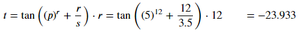Example 3: Quadratic equation with square root.

## Python3

 `# importing the module``import` `handcalcs.render``from` `math ``import` `sqrt`` ` `a ``=` `6``b ``=` `7``c ``=` `-``8`` ` `# run the code below in a new Jupyter cell``%``%``render``r ``=` `(``-``b ``+` `sqrt(b ``*``*` `2` `-` `4` `*` `a ``*` `c)) ``/` `(``2` `*` `a)`

Output: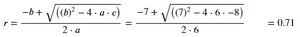### Comment Tags

By using comments, HandCalcs make some conclusions as to how the equation to be structured. Only a single comment can be used per cell.

Three types of customizations can be created using the # comment tags at the top of the cell:

1. # Parameters: The display structure of the variables or parameters can be controlled by using the # Parameter tag, this tag is used to classify the display structure into the vertical display or horizontal display.

Example: Without the # Parameter comment, all the equations will be displayed vertically

## Python3

 `# importing the module``import` `handcalcs.render`` ` `# run the code below in a new Jupyter cell``%``%``render``p ``=` `5``q ``=` `4``r ``=` `3``s ``=` `2``t ``=` `1`

Output: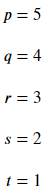Example: This time the # Parameter comment is used.

## Python3

 `# importing the module``import` `handcalcs.render`` ` `# run the code below in a new Jupyter cell``%``%``render`` ` `# Parameter``p ``=` `5``q ``=` `4``r ``=` `3``s ``=` `2``t ``=` `1`

Output: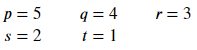2. # Long and # Short: As # Parameter comment tag is used to control the display structure of variables in the same way # Long and # Short comment tags control the display structure of equations, the # Long and # Short are used to display equations vertically and horizontally respectively.

Example: Displaying the equations horizontally using # Short.

## Python3

 `# importing the modules``import` `handcalcs.render``from` `math ``import` `sqrt`` ` `a ``=` `6``b ``=` `7``c ``=` `-``8`` ` `# run the code below in a new Jupyter cell``%``%``render`` ` `# Short``x ``=` `b ``*``*` `2` `-` `4` `*` `a ``*` `c``d ``=` `sqrt(x)``r1 ``=` `(``-``b ``+` `d) ``/` `(``2` `*` `a) ``r2 ``=` `(``-``b ``-` `d) ``/` `(``2` `*` `a)`

Output: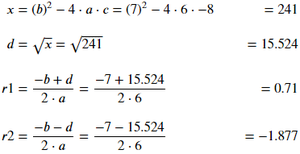Example: Displaying the equations vertically using # Long.

## Python3

 `# importing the modules``import` `handcalcs.render``from` `math ``import` `sqrt`` ` `a ``=` `6``b ``=` `7``c ``=` `-``8`` ` `# run the code below in a new Jupyter cell``%``%``render`` ` `# Long``x ``=` `b ``*``*` `2` `-` `4` `*` `a ``*` `c``d ``=` `sqrt(x)``r1 ``=` `(``-``b ``+` `d) ``/` `(``2` `*` `a) ``r2 ``=` `(``-``b ``-` `d) ``/` `(``2` `*` `a)`

Output: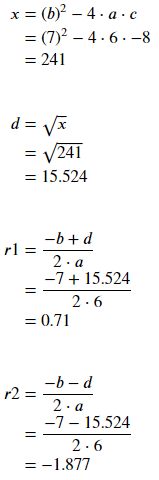3. # Symbolic: The HandCalcs library’s primary objective is to make the complete equation using the numerical substitution. This makes the equation easy to track and validate. However, there might be situations where equations are represented symbolically, the # Symbolic comment tag cab symbolically render Latex equations.

Example:

## Python3

 `# imporintg the modules``import` `handcalcs.render``from` `math ``import` `sqrt, tan`` ` `# Parameters``a ``=` `6``b ``=` `7``c ``=` `-``8``x ``=` `9``y ``=` `10`` ` `# run the code below in a new Jupyter cell``%``%``render`` ` `# Symbolic``r ``=` `(``-``b ``+` `sqrt(b ``*``*` `2` `-``4` `*` `a ``*` `c)) ``/` `(``2` `*` `a)``z ``=` `tan(x ``*``*` `y ``+` `y ``/` `x)`

Output: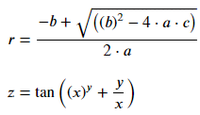My Personal Notes arrow_drop_up